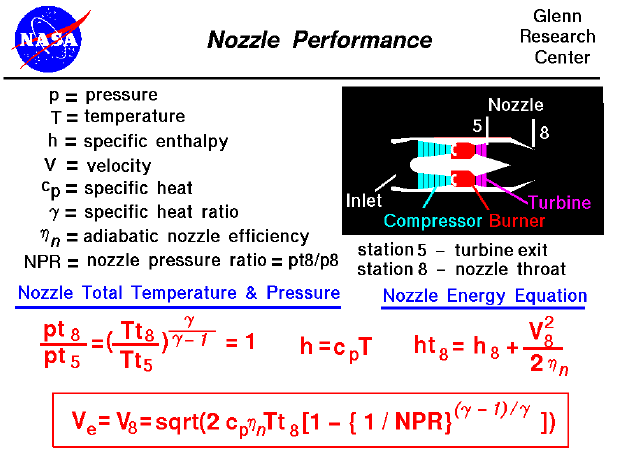A text only version of this slide is available which gives all of the flow equations. The interactive Java applet EngineSim is also available. This program solves these equations and displays the thrust and fuel flow values for a variety of turbine engines.

Most modern passenger and military aircraft are powered by gas turbine engines, which are also called jet engines. There are several different types of jet engines, but all jet engines have some parts in common. All jet engines have a nozzle which produces the thrust as described on the thrust equation slide. The nozzle also sets the total mass flow rate through the engine as described on a separate slide. The nozzle sits downstream of the power turbine and, while the nozzle does no work on the flow, there are some important design features of the nozzle. Because the nozzle does no thermodynamic work, the total temperature (Tt) through the nozzle is constant. Referring to our station numbering, the nozzle entrance is station "5" and the nozzle exit is station "8". Tt8 divided by Tt5 is 1.0 as shown on the slide. The total pressure (pt) across the nozzle is constant as well (pt8 / pt5 = 1.0). The static pressure at the exit of the nozzle is equal to free stream static pressure, unless the exiting flow is expanded to supersonic conditions. (A convergent-divergent nozzle will have supersonic exit flow, a simple convergent nozzle will not.) The ratio of the nozzle total to static pressure ratio is called the nozzle pressure ratio (NPR).

Considering the energy equation for the nozzle, the specific total enthalpy is equal to the static enthalpy plus the square of the exit velocity divided by two. An efficiency factor has been included here to account for all the losses in the nozzle, but its value is normally very near 1.0. With a little algebra (which you learned in middle school), and using the definitions shown on the slide, you can solve the energy equation for the exit velocity. The answer is shown in the red box. Using this velocity, and the mass flow rate through the engine, we can solve the thrust equation for the amount of thrust produced by the nozzle.

As shown on this slide, the exit velocity depends on the nozzle pressure ratio and the nozzle total temperature. The nozzle pressure ratio depends on the exit static pressure (which we know) and the nozzle total pressure. We can determine the nozzle total pressure from the free stream conditions and the engine pressure ratio (EPR). EPR depends on the pressure ratio of all the other engine components. We can also determine the nozzle total temperature from the engine temperature ratio (ETR). ETR depends on the temperature ratio of all the other engine components. With this information, we can solve for the thrust developed by a jet engine.

The nozzle performance equations work just as well for rocket engines except that rocket nozzles always expand the flow to some supersonic exit velocity. You can explore the design and operation of turbojet and rocket nozzles with our interactive nozzle simulator program which runs on your browser.Guided Tours